DDG（离散微分几何）：拉普拉斯 | HyperPlane

## 基本性质

$\Delta \phi = \rho$

$\langle f, g \rangle \coloneqq \intop_\Omega f(x)g(x) dx$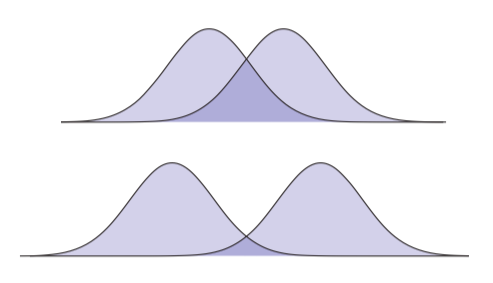$\langle X, \Upsilon \rangle \coloneqq \intop_\Omega X(x) \cdot \Upsilon(x)dx$

$\langle \Delta f, g\rangle = -\langle \nabla f, \nabla g \rangle + \langle N \cdot \nabla f, g \rangle_\partial$

$\langle \Delta \phi, \phi \rangle \geq 0$

（顺便，为什么这个值不是严格大于0的呢？）语言无法解释半正定的重要性。让我们考虑一个非常简单的例子：在平面中形式为$\phi(x,y) = ax^2 + bxy + cy^2$的函数。任意一个这样的函数可以写为矩阵形式：

$\phi(x,y) = \underbrace{\begin{bmatrix} x & y \end{bmatrix}}_{\mathbf{x}^T} \underbrace{\begin{bmatrix} a & b/2 \\ b/2 & c \end{bmatrix}}_A \underbrace{\begin{bmatrix} x \\ y \end{bmatrix}}_{\mathbf{x}} = ax^2 + bxy + cy^2$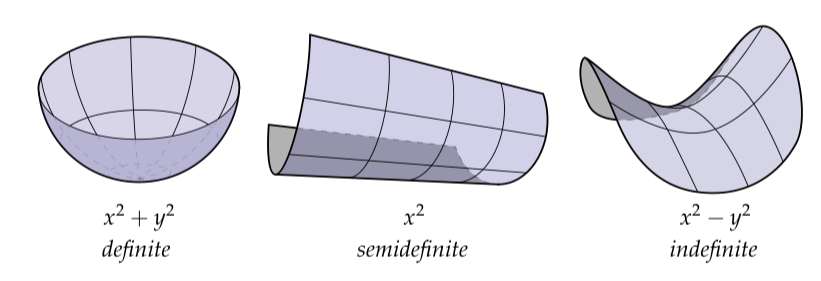## 通过有限元方法离散化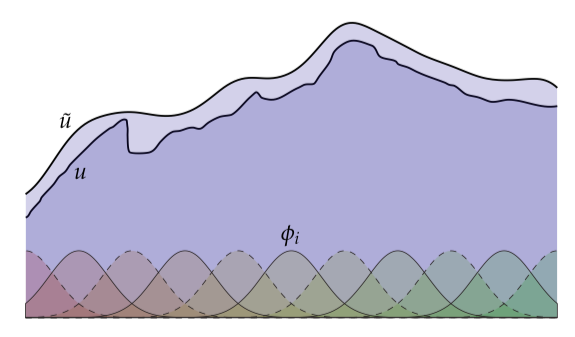$\tilde{u} = \sum_i x_i \phi_i, x_i \in \mathbb{R}$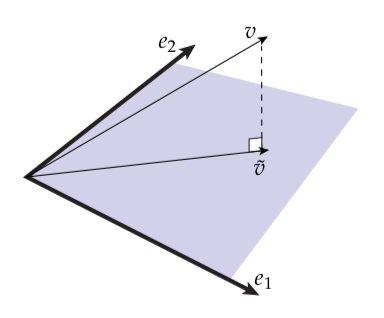\begin{aligned} (\tilde{v} - v) \cdot e_1 &= 0 \\ (\tilde{v} - v) \cdot e_2 &= 0 \end{aligned}

$\Delta u = f$

$\langle \Delta \tilde{u} - f, \phi_j \rangle = 0$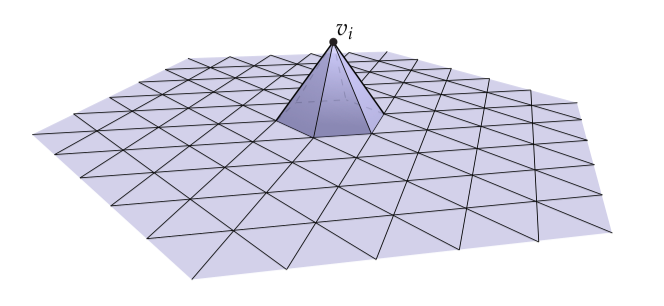\begin{aligned} \langle \Delta u, \phi_j \rangle &= \sum_k \langle \Delta u, \phi_j \rangle_{\sigma_k} \\ &= \sum_k \langle \nabla , \nabla \phi_j \rangle_{\sigma_k} + \sum_k \langle N \cdot \nabla u, \phi_j \rangle_{\partial \sigma_k} \end{aligned}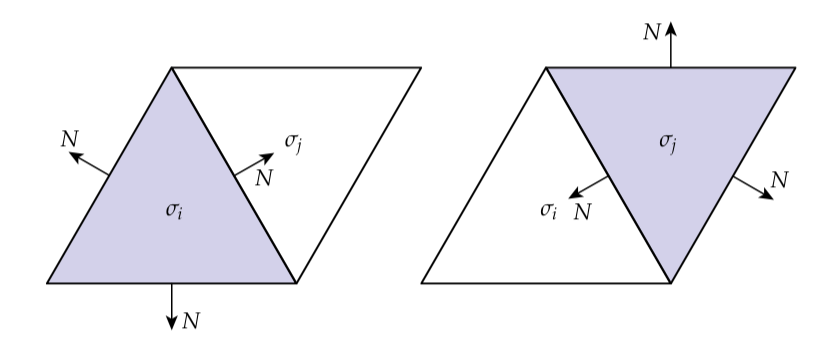$\langle \nabla u, \nabla \phi_j \rangle$

$\langle \nabla u, \nabla \phi_j \rangle = \langle \nabla \sum_i x_i \phi_i, \nabla \phi_j \rangle = \sum_i x_i \langle \nabla \phi_i, \nabla \phi_j \rangle$

$A_{ij} \coloneqq \langle \nabla \phi_i, \nabla \phi_j \rangle$

$Ax = b$

$(\Delta u)_i = \frac{1}{2} \sum_j (\cot \alpha_j + \cot \beta_j)(u_j - u_i)$

## 通过离散外微积分方法离散化

FEM的方式显示了一种相当标准的做法去离散化偏微分方程。但是让我们尝试一个不同的方法，基于离散外微积分（DEC）。相当有趣的是，虽然这两个方式十分不同，但是我们最后会得到一样的结果！

$\star d \star d u = f$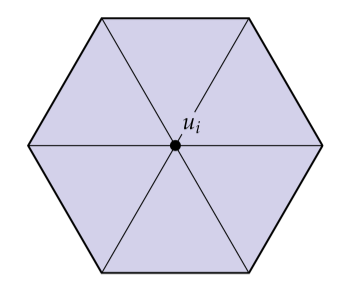$(du)_{ij} = \intop_{e_{ij}} du = \intop_{\partial e_{ij}} u = u_j - u_i$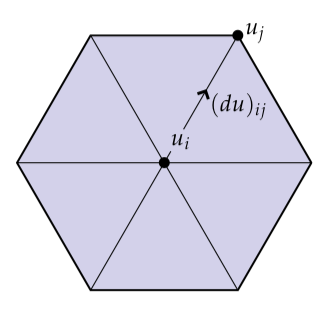（注意到边的边界$\partial e_{ij}$就是两个端点$v_i$$v_j$。）Hodge星将沿着边$e_{ij}$的环流转变为穿过对应对偶边$e^{\star}_{ij}$的通量。特别的，我们拿着沿着原始边的全部环流，将它除以边的长度得到平均逐点环流（average pointwise circulation），然后乘上对偶边的长度得到通过对偶边的全部通量：

$(\star du)_{ij} = \frac{|e^{\star}_{ij}|}{|e_{ij}|}(u_j - u_i)$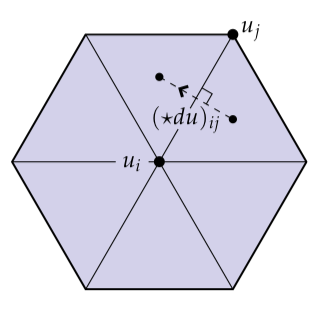$(d \star du)_i = \intop_{C_i}d \star du = \intop_{\partial C_i} \star du = \sum_j \frac{|e^{\star}_{ij}|}{|e_{ij}|}(u_j - u_i)$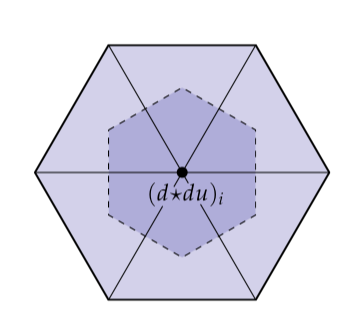$(\star d \star du)_i = \frac{1}{C_i}\sum_j \frac{|e^{\star}_{ij}|}{|e_{ij}|}(u_j - u_i) = f_i$

$d \star du = \star f$

## 网格和矩阵

$(\Delta u)_i = \frac{1}{2}\sum_j (\cot \alpha_j + \cot \beta_j) (u_j - u_i)$

$\Delta u = f$

$(Bu)_i = \sum_ju_j$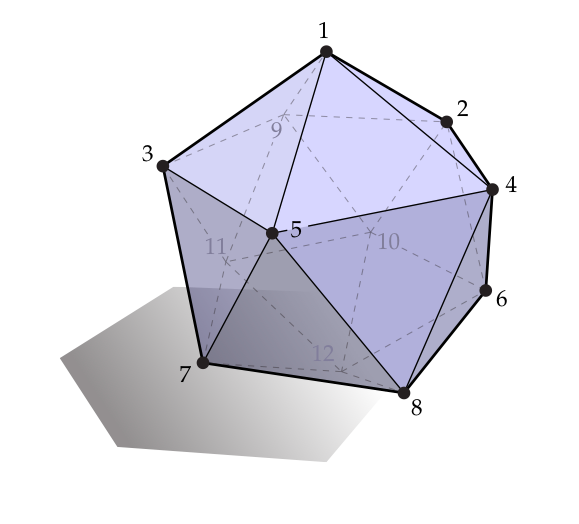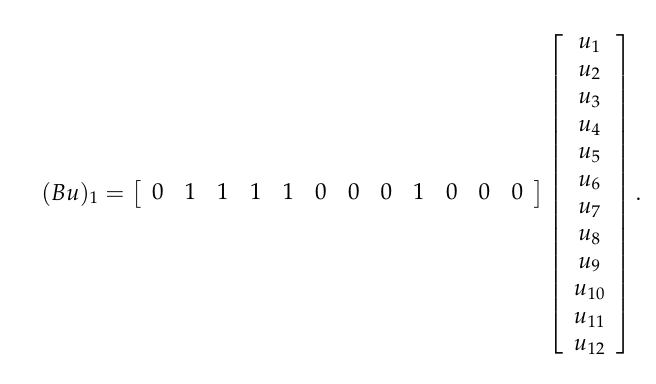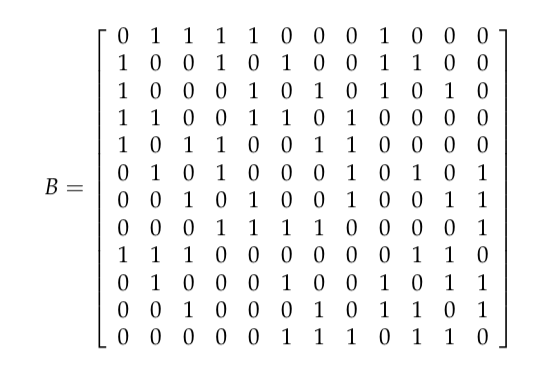（你可以验证这个矩阵是对的，或者你可以出去晒晒太阳，这是你的选择。）实际上，幸运的是，我们不必“手动“构建矩阵——我们可以简单的开始于0矩阵然后通过循环我们网格的局部邻域去使用非0元素填充它。